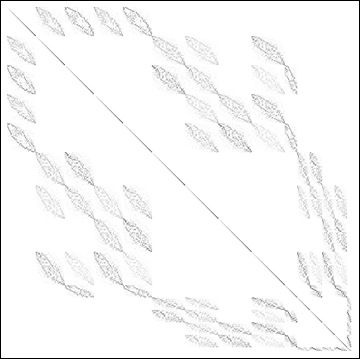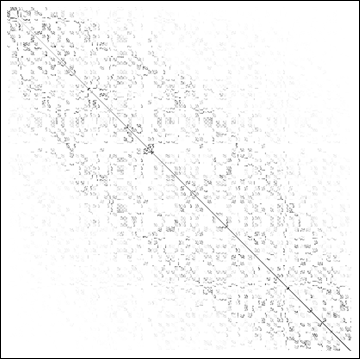# Numerical renormalization group

Numerical renormalization group (NRG) is a numerical non-perturbative approach to the renormalization group for many-particle problems, as devised by Kenneth Wilson. It is the most accurate method known to handle the quantum impurity problems, such as the Kondo problem and the Anderson impurity problem. Such models describe magnetic impurities in interaction with itinerant conduction band electrons in a metal. The impurity local moment (spin) couples antiferromagnetically to the conduction electrons, which leads to the screening of the spin at low temperatures (on the scale of the Kondo temperature, TK).

The method consists of the following main elements:

• Reduction to a one-dimensional problem: if an impurity is isotropic, it only couples to conduction electron states that are, likewise, isotropic around the impurity (the s waves). Conduction states with other symmetries (p, d, ...) are in this approximation irrelevant. The s waves form a one parameter set (indexed by the wave number k or energy ω). The prototype Kondo problem is thus a one-dimensional field theory.
• Logarithmic discretization of the conduction band: the effective one-dimensional conduction band still has an infinite number of degrees of freedom (a countable continuum for a system of finite size). For numerical calculation, the number of states needs to be reduced to a manageable amount. In NRG, this is achieved by logarithmically discretizing the conduction bands: the band is divided into intervals with geometrically decreasing widths Λ-N, where Λ is a discretization parameter, Λ>1. Each interval is then Fourier transformed and only the zero (constant) mode in each interval is retained. It was shown by Wilson that the neglect of higher Fourier modes leads to a small error, which can in principle be systematically decreased by reducing Λ towards 1; this corresponds to going back to the continuum limit.
• Tridiagonalisation and the (Wilson) chain Hamiltonian: the Hamiltonian that describes the impurity and the retained states in the discretized conduction band can be cast in the form of a one-dimensional tight-binding Hamiltonian where each site couples only to the nearest neighbors with exponentially decreasing matrix elements. The topology of inter-site couplings is that of a chain, and the Hamiltonian is sometimes named the Wilson chain Hamiltonian.
• Iterative diagonalization: the resulting chain Hamiltonian is then diagonalized iteratively. One starts by exactly diagonalizing the impurity site and (optionally) the first few sites of the chain. One then adds one site at a time, couples it to the eigenstates of the previous NRG iteration and performs a new diagonalization. Due to exponentially decreasing coupling matrix elements, this corresponds to considering excitations on a reduced energy scale, given approximately by Λ-N/2.
• Truncation of states: since, with each added site of the Wilson chain, the number of states grows by a factor of 4, the resulting matrices become of unmanageable size in just a few iterations. For this reason, after each iteration we need to truncate the number of states, i.e. we keep only a few 1000's of states with the lowest energies. Alternatively, we keep states of (rescaled) energies below a certain arbitrary cutoff energy. Wilson had shown that the error introduced by this procedure in the following iteration is small for the excitations with the lowest energies, which are precisely those states that determine the physical properties. By increasing the number of states retained, the error can be systematically reduced. The smaller the discretization parameter Λ is, the more states we need to retain. In practical terms this means that a compromise must be made between the discretization error and the truncation error, since a small Λ would require retaining too many states, while a large Λ increases discretization errors. With increasing performance of modern computers, the complexity of the problems that can be efficiently computed grows. It is now possible to accurately calculate three-impurity two-channel problems on a modest cluster of computers.

•Typical matrix structure of the Hamiltonian in some invariant subspace. The example is taken from a calculation for a two-channel spin-1/2 Kondo model using SU(2) spin and SU(2) isospin symmetry. The block structure of the matrix has its origin in the coupling of 16 invariant subspaces from the previous iteration step via the newly added chain site.If the Hamiltonian matrix is reordered so that the diagonal matrix elements are sorted in increasing order, the previous matrix takes a very characteristic form with matrix elements that are decreasing as we move away from the matrix diagonal. This is an explicit manifestation of the energy scale separation, which is the particular property of quantum impurity problems that makes NRG calculations successful!